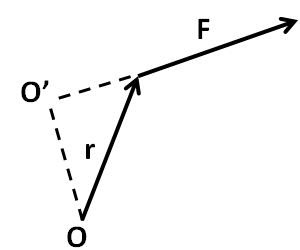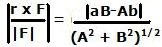Monday, November 10, 2008

Irodov Problem 1.235The moment of force (torque) N is given by the vector cross product r x F computed as,The moment arm is the length of the projection of vector r on the perpendicular to vector F (the length OO') and is given by,